# How to Count Cells in Google Sheets

When you’re compiling a spreadsheet in Google Sheets, you might need to know the number of certain cells. Different Google Sheets functions and formulas are typically used to count blank and non-blank cells. This task might sound complicated for first-time Google Sheets users, but once you get the hang of it, you’ll see that counting cells in this online program is just a matter of designated steps.In this article, we’ll show you how to count various types of cells in Google Sheets, including text cells, selected cells, filtered cells, and more.

## How to Count Cells in Google Sheets

If your Google Sheets document only has a few columns and rows, you can always count the number of cells manually. However, if the spreadsheet contains a large database, knowing how to use formulas to count cells in Google Sheets can save you a lot of time.

To count cells in Google Sheets, we’ll be using a few special formulas. Specifically, COUNT functions are used to count the number of cells that are within a specific data range. The two most common and useful types of COUNT functions in Google Sheets are COUNTA and COUNTIF.

The COUNTA formula indicates the number of numeric values within one dataset. The COUNTIF formula is used to determine a conditional count across a range. It’s written like this: COUNTIF (range, criterion), where the range is tested against the criterion. Sometimes you can use the SUMPRODUCT formula, which shows the sum of products of corresponding ranges in two arrays.

## How to Count Cells in Google Sheets by Color

There is no direct way to count the number of cells based on the background color. Instead, you will have to use a custom function with Google Apps Script.

First, you will need to copy this code.

“function countColoredCells(countRange,colorRef) {

var activeformula = activeRg.getFormula();

var countCells = 0;

for (var i = 0; i < backGrounds.length; i++)

for (var k = 0; k < backGrounds[i].length; k++)

if ( backGrounds[i][k] == BackGround )

countCells = countCells + 1;

return countCells;

};”

Here’s how you can use this code to count cells in Google Sheets by color.2. Find the spreadsheet you want to edit.3. Navigate to “Extension” on the menu and then to “Apps Script.”4. Delete the code in the Google Apps Script window.5. Paste the code provided above.6. Save the changes by clicking on the disc icon at the top of the page.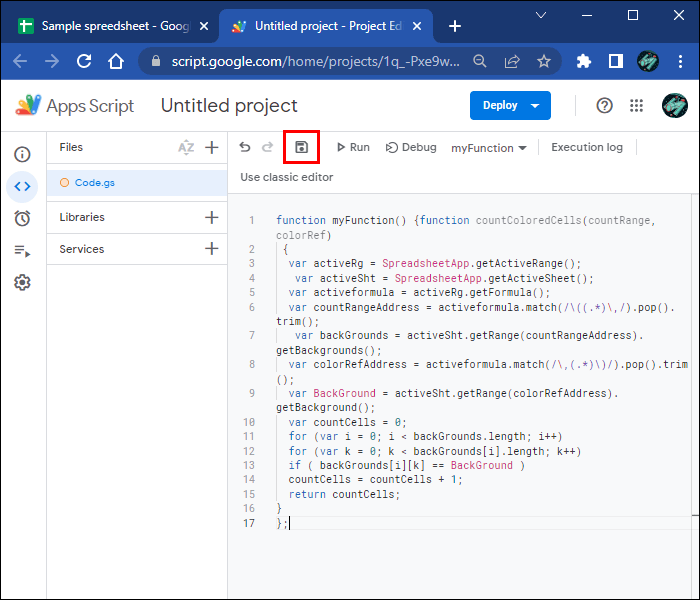7. Close the Google Apps Script window.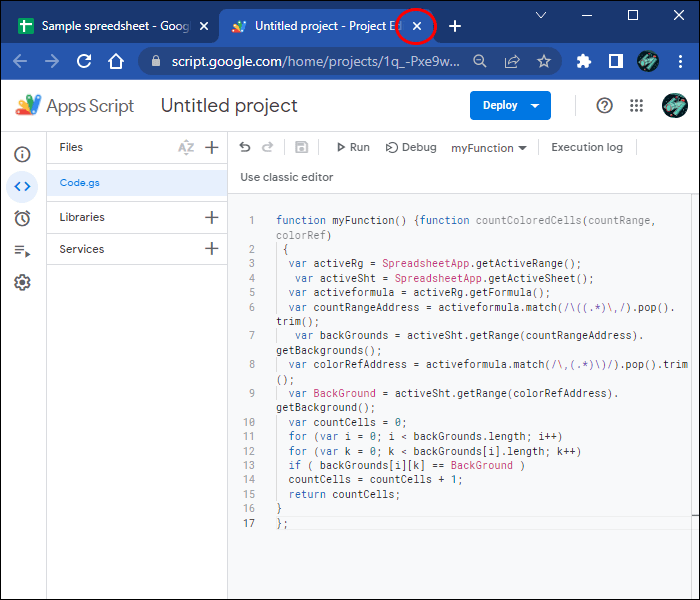8. Enter “=COUNTCOLOREDCELLS” in any empty cell in the spreadsheet.9. Write the range in parentheses, like in this example: “=COUNTCOLOREDCELLS (A12:D15)”.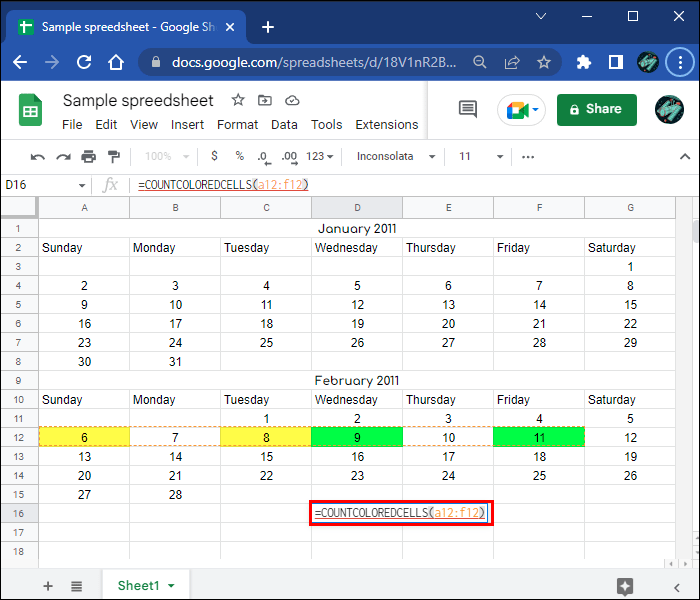10. Press “Enter” on your keyboard.

The number of colored cells will appear in the cell where you entered the formula.

## How to Count Text Cells in Google Sheets

In most cases, you will be required to count cells with text. It doesn’t matter what kind of text the cells contain; it could be numbers, dates, names, etc. Note that there is a difference between counting cells with specific and non-specific text. We will be using the COUNTIF formula for both.

To count non-specific text cells in Google Sheets, this is what you need to do.2. Locate the spreadsheet you want to use.3. Double-click on an empty cell where the COUNT results will be.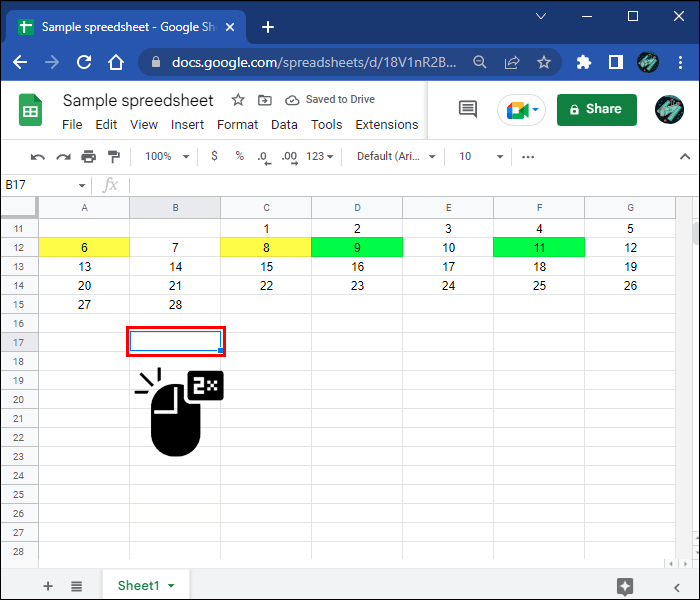4. Type in the “=COUNTIF” formula in the selected empty cell, or just choose it from the suggestions list.5. Select the text cells you want to count by dragging your mouse over the range. It will be
automatically added to the COUNTIF formula. For example: “=COUNTIF (B4:C7” is what you’re supposed to write for now (no closing parenthesis yet).
Note: Another way to do this is by manually typing the range after the opening parenthesis. For example, “(A7:C11.”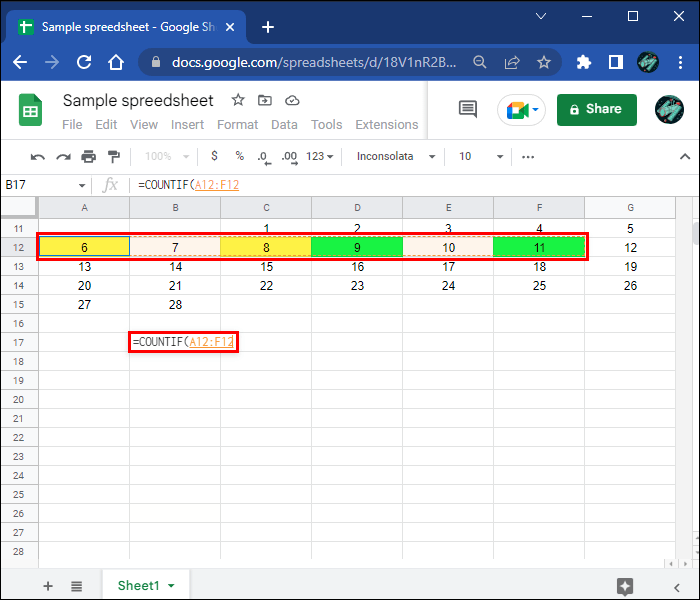6. Write the qualifying criterion in quotation marks (e.g., “>20”). This is the only time we will be using quotation marks in the formula. The criterion you use depends on the cells’ content.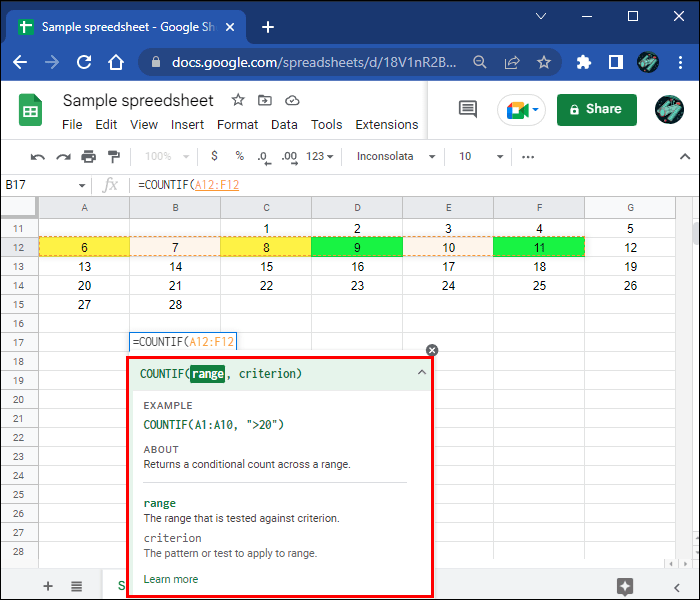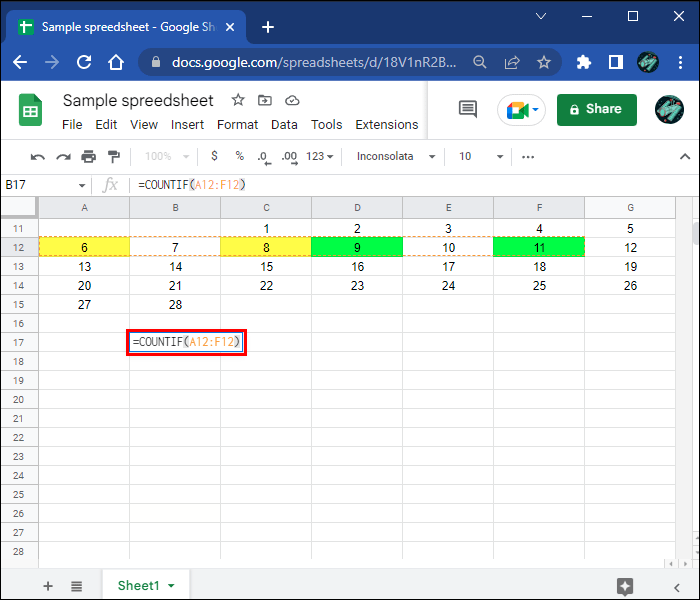8. Press the “Enter” key.

The number of text cells will appear in the cell where you entered the formula

## How to Count Selected Cells in Google Sheets

To count the number of selected cells in Google Sheets, you can use the COUNTA formula. This is how it’s done.2. Find the spreadsheet you want to use.3. Double-click on any empty cell in the spreadsheet.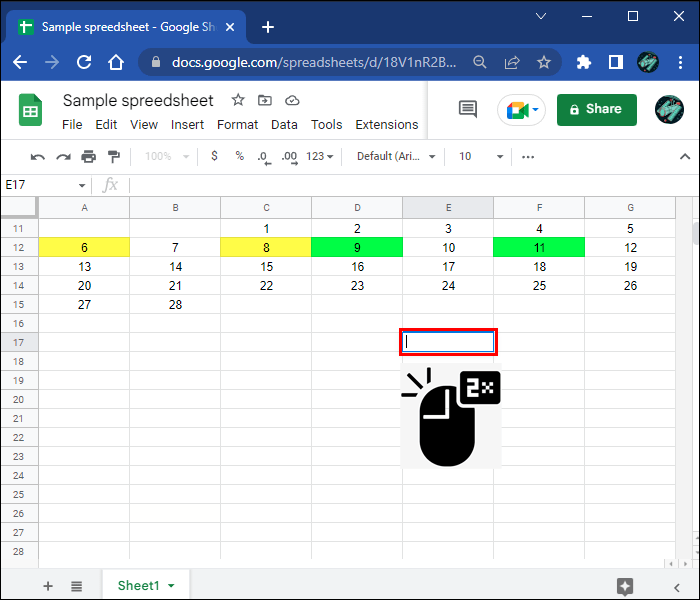4. Write “=COUNTA” in the cell.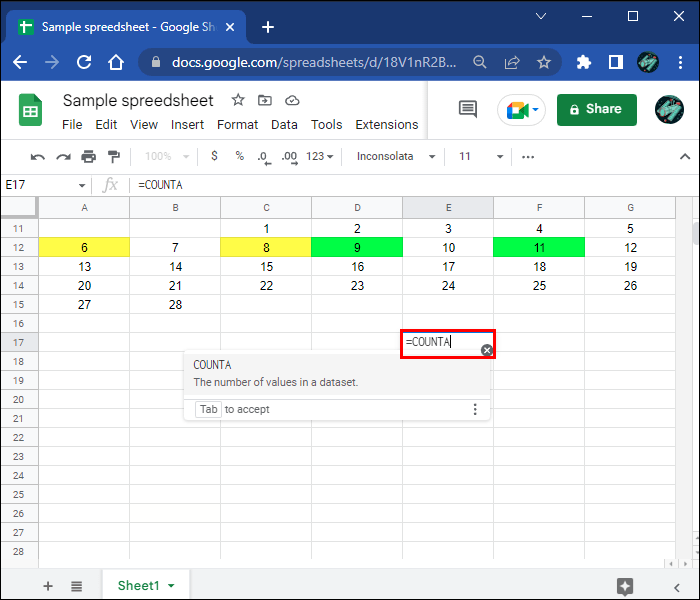5. Select the cells you want to count by clicking on the range and dragging the cursor over it. You can also just enter the range in the parentheses, as in the following example: “=COUNTA (A1:B10).”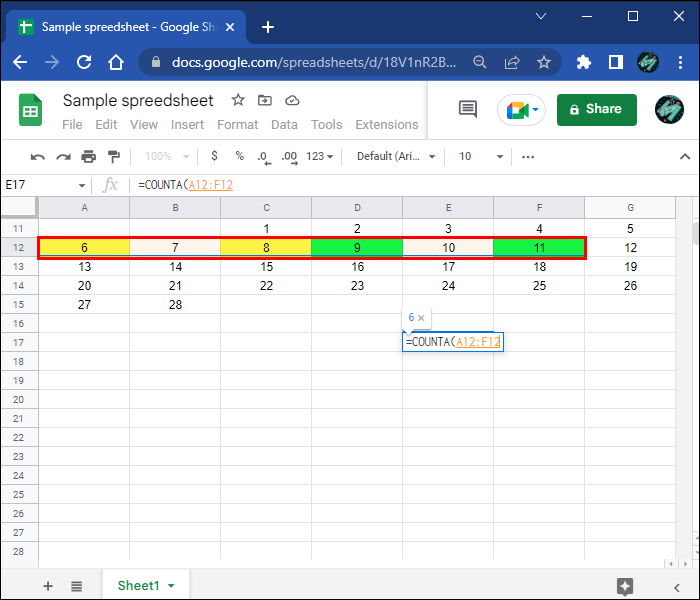6. Press “Enter” on your keyboard.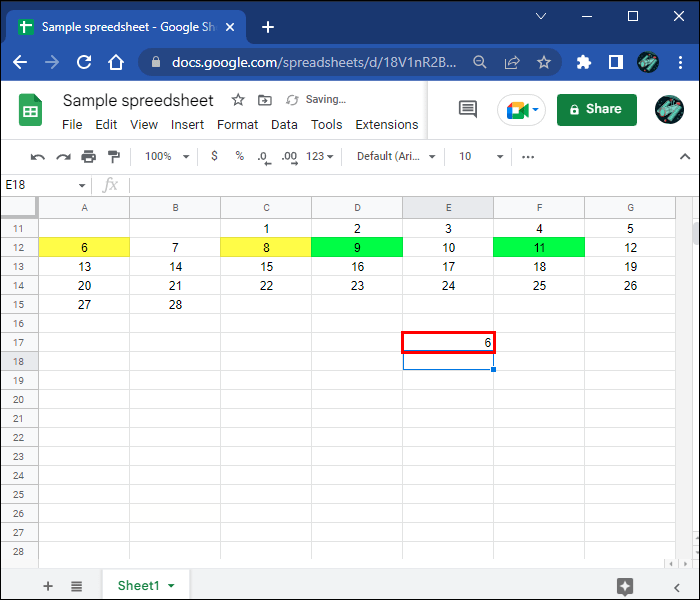You’ll see the number of selected cells in the same cell where you wrote the formula. We won’t be using quotation marks in the COUNTA formula.

## How to Count Filtered Cells in Google Sheets

If you want to count cells with specific words, you can use the COUNTIF formula. For example, if you want to count all the cells that contain the word “product,” the COUNTIF formula is ideal for this. Follow the steps below to see how it works.2. Double-click anywhere on the spreadsheet.3. Write “=COUNTIF.”4. In parentheses, add the range. For example: “(A3:B10)”.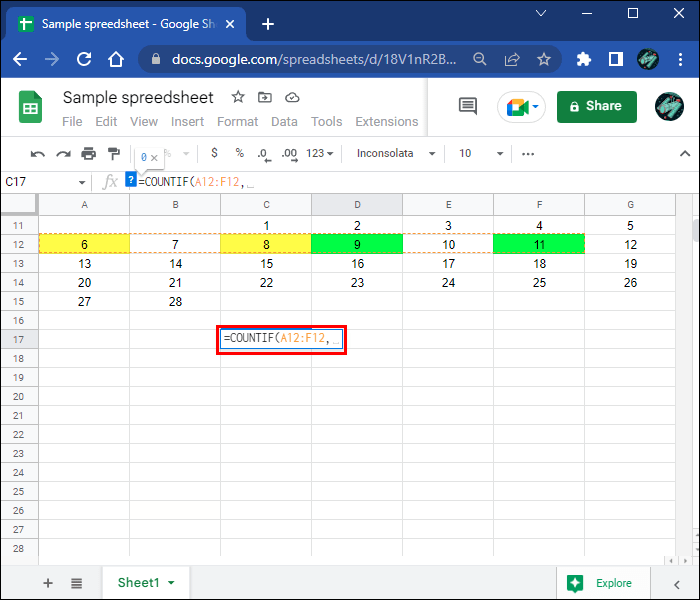6. Enter the filter word between quotation marks. This is what the formula is supposed to look like “=COUNTIF (A3:B10, “product”).”7. Press “Enter.”

## How to Count Bold Cells in Google Sheets

Just like with colored cells, there is no function you can use to count the number of bold cells in Google Sheets. You will need to use a custom formula using Google Apps Script. Here’s how it’s done.2. Navigate to “Extension” on the top menu.3. Select “Apps Script” from the list of options. This will take you to Google Apps Script.4. Delete the code that’s already there.5. Copy and paste the following formula in the Code.gs window.
“function countboldcells() {
var sheet = book.getActiveSheet();
var range_input = sheet.getRange(“E2:S7”);
var range_output = sheet.getRange(“G14”);
var cell_styles = range_input.getFontWeights();
var count = 0; for(var r = 0; r < cell_styles.length; r++) {
for(var c = 0; c < cell_styles.length; c++) {
if(cell_styles[r][c] === “bold”) {
count = count + 1;
}
}
}
range_output.setValue(count)
}”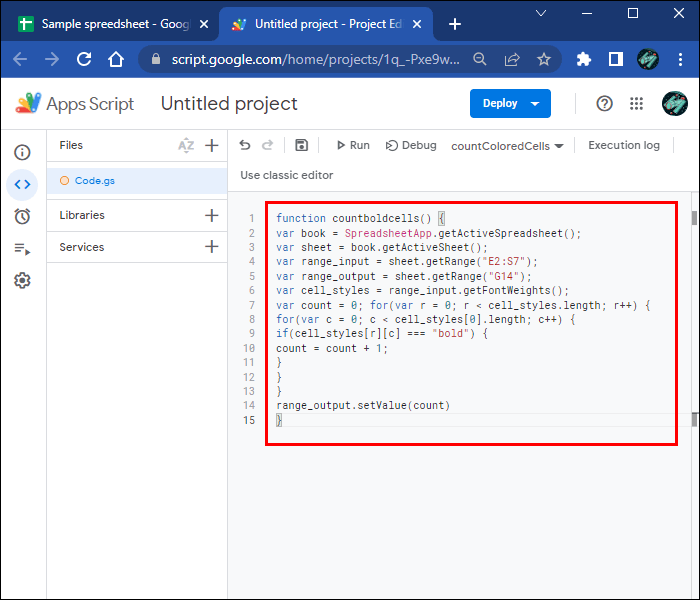6. Select the “Save” icon above the code.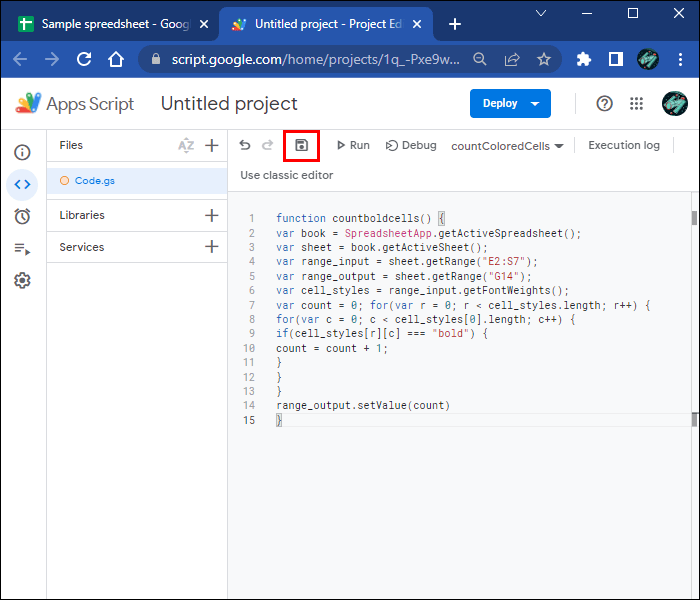Now that you’ve added the ability to count bold cells in Google Sheets, this is what you should do next.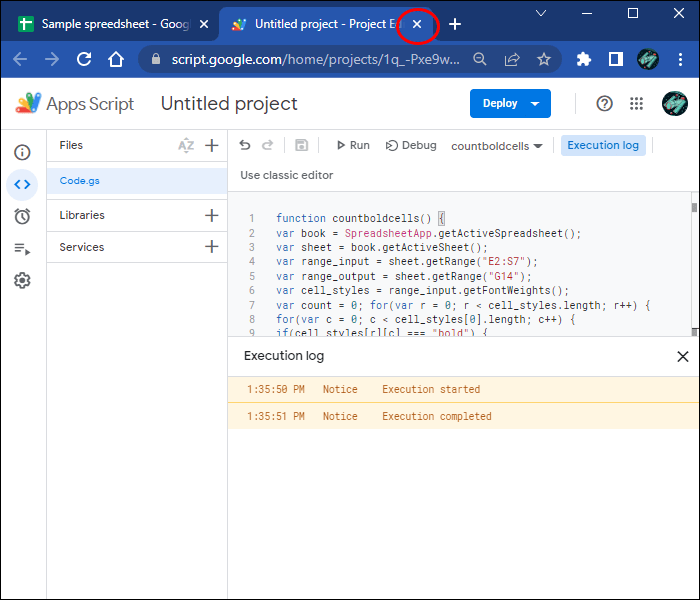8. Write “=COUNTBOLDCELLS” in an empty cell.9. Enter the range, for example: “(A1:B10)”.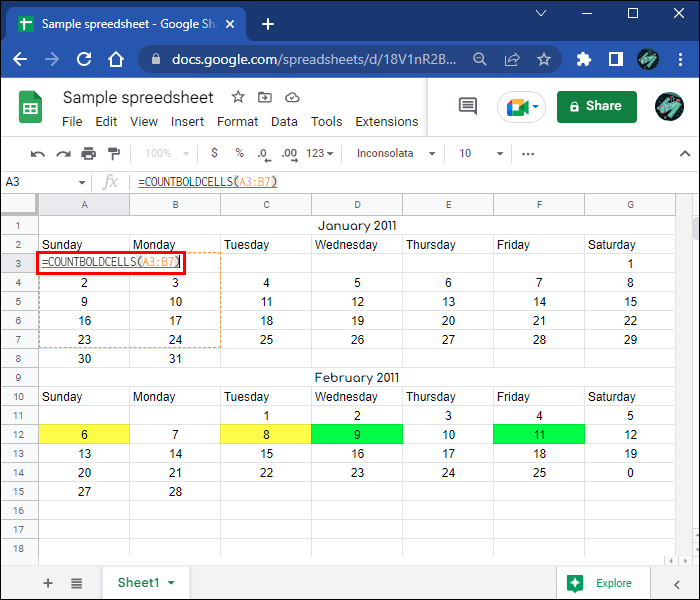10. Press the “Enter” key.

All the bolded cells will be counted, and the results will show up in that cell.

## How to Count Blank Cells in Google Sheets

Google Sheets lets you count cells that don’t contain any text. These are called blank cells. We will count them using the COUNTBLANK formula. This is how it’s done.2. Choose any empty cell in the spreadsheet and double-click on it.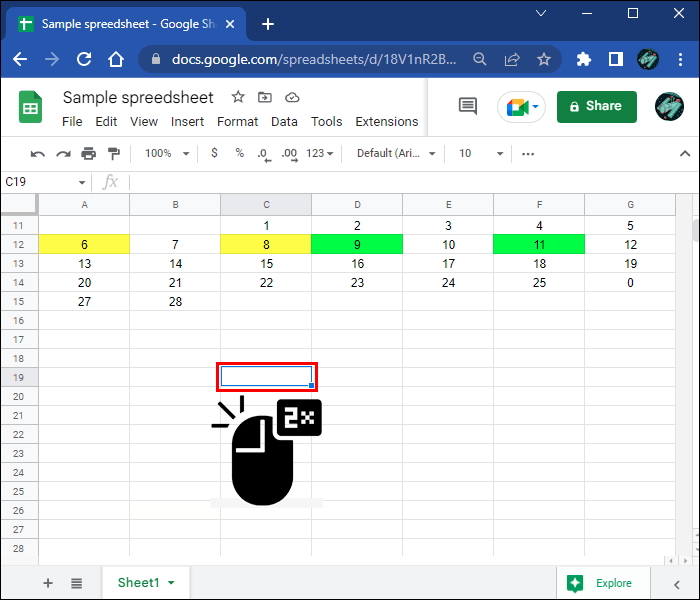3. Enter “=COUNTBLANK” in the empty cell.4. Type the cell range in parentheses, as in the example: “=COUNTBLANK (A:D)”.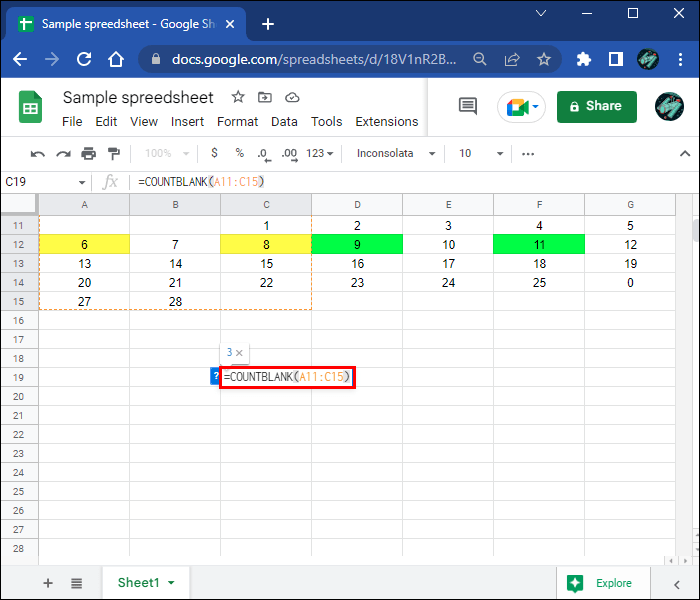5. Press “Enter.”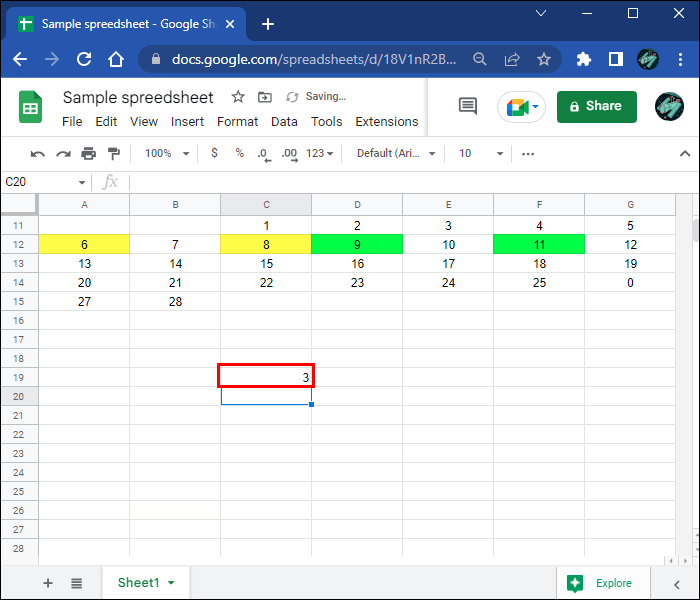That’s all there is to it. The number of blank cells will appear in that cell.

Keep in mind that Google Sheets differentiates blank and empty cells. If there’s an empty text string in a cell, Google Sheets sees it as an empty cell, not a blank one.

## How to Count Non-Blank Cells in Google Sheets

To count non-blank cells in Google Sheets, we’ll be using the COUNTA function. This function is used to count cells that contain a text string or some kind of value within a range. If there are blank cells between text cells, they won’t be picked up by the COUNTA function.2. Choose an empty cell anywhere on the spreadsheet.3. Enter “=COUNTA” in the empty cell.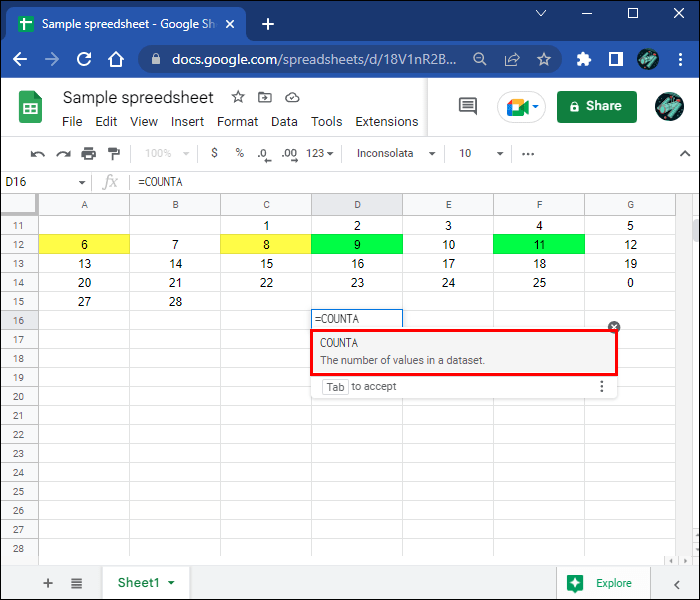4. Type the cell range in parentheses, for example: “(B5:B20)”.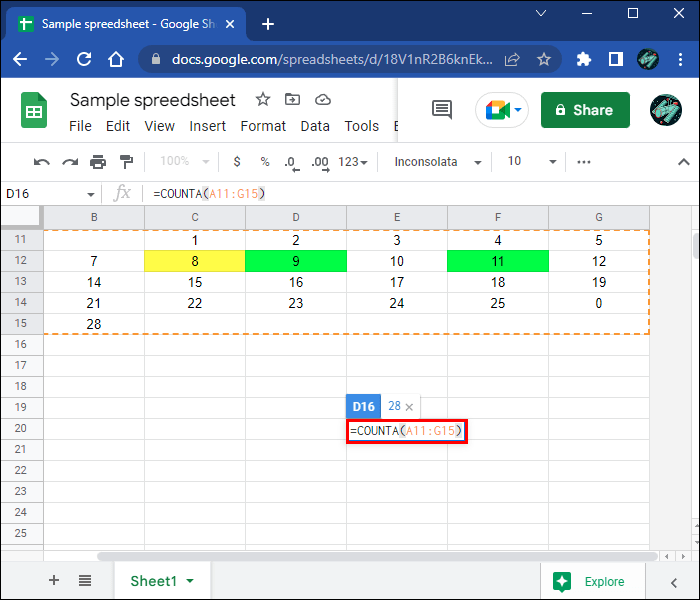5. Press “Enter” on your keyboard.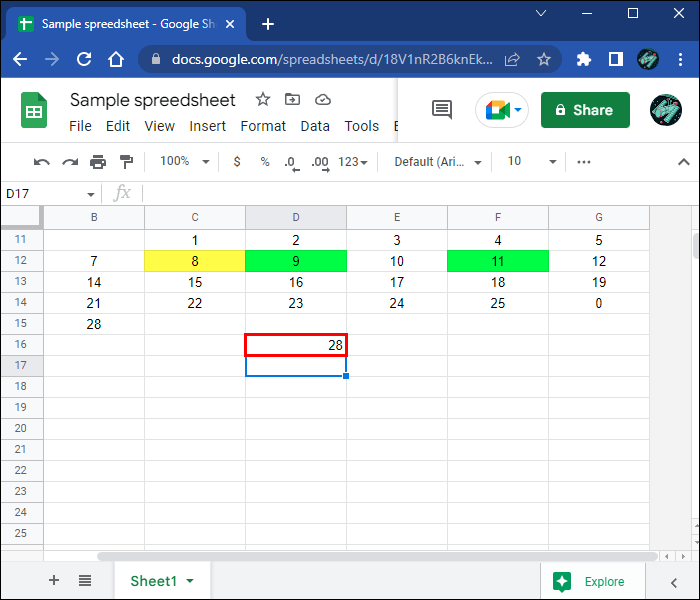The number of non-blank cells within a range will appear in the cell. If your spreadsheet has hidden or special characters, the COUNTA function can’t help you. In these cases, it’s best to use the COUNTIF or the SUMPRODUCT function.

## Count Cells Like a Pro

Using Google Sheets might be complicated at first, especially if you’ve never used its formulas before. If you’re required to count specific cells in your spreadsheet, there’s probably a formula that can help you. If not, you will have to use a custom formula with Google Apps Script.

Have you ever counted cells in Google Sheets? Which formula did you use? Let us know in the comments section below.

Disclaimer: Some pages on this site may include an affiliate link. This does not effect our editorial in any way.

Todays Highlights